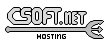Agar 1.7 Manual

M_Plane(3)

# SYNOPSIS

```#include <agar/core.h>
#include <agar/gui.h>
#include <agar/math/m.h>
```

# DESCRIPTION

The M_Plane structure describes a plane in R3 (Ax+By+Cz+D=0):
```typedef struct m_plane {
M_Vector3 n;		/* Normal vector */
M_Real d;		/* Distance to origin */
} M_Plane;
```

The plane satisfies the equation A(n.x) + B(n.y) + C(n.z) = d.

# INITIALIZATION

M_Plane M_PlaneFromPts (M_Vector3 p1, M_Vector p2, M_Vector p3)

M_Plane M_PlaneFromParallel (M_Plane P, M_Real d)

void M_PlaneWrite (AG_DataSource *ds, M_Plane *P)

M_Plane M_PLANE_INITIALIZER (M_Real a, M_Real b, M_Real c, M_Real d)

The M_PlaneFromPts() function returns a plane from three (non-colinear) points p1, p2 and p3. M_PlaneFromParallel() returns the parallel plane at distance d from the specified plane P.

The M_PlaneRead() and M_PlaneWrite() functions read or write a plane structure from/to an AG_DataSource(3).

The macro M_PLANE_INITIALIZER() expands to a static initializer for a M_Plane from the coefficients of the plane equation a, b, c, d.

# TESTS

M_Real M_PlaneVectorAngle (M_Plane P, M_Vector3 v)

The M_PlaneVectorAngle() function computes the angle between the plane and specified vector, in radians.www.libAgar.org is © 2022 Julien Nadeau Carriere <vedge@csoft.net>.